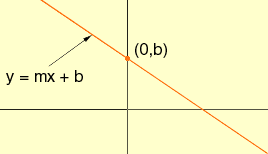what is slope intercept form? Hi, The slope intercept form is one of the ways of writing the equation of a line. This form uses the slope of the line, usually designated m, and the y-coordinate of the point where the line intersects the y-axis. This point is usually designated (0,b). The slope intercept form of this line is then y = mx + b.Cheers, Penny Go to Math Central